# AP Chemistry : Reaction Rate and Rate Law

## Example Questions

← Previous 1

### Example Question #1 : Reaction Rate And Rate Law

In a third order reaction with two reactants, if you triple the concentration of one of the reactants, the rate increases by a factor of 3. What happens to the rate of the reaction if the concentration of the second reactant is halved?

It increases by a factor of 4.

It decreases by a factor of 4.

It doubles.

It is halved.

It decreases by a factor of 4.

Explanation:

Based on the question, you can tell that the rate is first order with respect to the first reactant. So if the overall reaction is third-order, that means that the exponents must add to 3. We know that the exponent of the first reactant is 1, so that must mean that the exponent of the second reactant is 2. Thus, the concentration will be squared when you account for how it contributes to the rate, so the rate increases by a facotor of 4.

### Example Question #1 : Reaction Rate And Rate Law

Given the following. What is the rate law for the reaction A + 2B → C

[A](M)         [B] (M)          Rate (M/s)

0.1               0.02             0.005

0.2               0.02             0.02

0.1               0.01             0.0025

Rate = k [B]

Rate = k [A]

Rate  = k [A] [B]2

Rate = k [A] [B]

Rate = k [A]2[B]

Rate = k [A]2[B]

Explanation:

The rate is only based on the experimental values in the table. When B doubles and A stays the same the rate doubles, making it first order with respect to compound B. When compound A doubles and B stays the same, the rate quadruples, making it second order in regards to A.

### Example Question #3 : Reaction Rate And Rate Law

The rate of the reaction 2A + B → C was measured at different concentrations of reactants. The results are as follows:

[A]    [B]      Rate

0.5    2        0.1

0.5    4        0.4

1       2        0.2

r = k[B]

r = k[A][B]2

r = k[A]

r = k[A][B]

r = k[A]2[B]

r = k[A][B]2

Explanation:

When the concentration of A stays the same but the concentration of B doubles, the rate quadruples, showing a second order rate law based on B. When the concentration of A doubles and B stays the same, the rate also doubles, showing a first order rate law based on A.

### Example Question #1 : Reaction Rate And Rate Law

A rate law __________.

is measured in unit of concentration over time

can always be derived from looking at the reaction in question

can never be completely independent of the concentrations of any of the reactants

always requires experimental data to determine

is measured in unit of concentration over time

Explanation:

Rate laws can rarely be determined from just the reaction; they usually require experimental data. However, both of the answer choices contain the word ALWAYS, which is too extreme. Rate laws can be independent of the reactants, these rate laws are known as zero-order.

### Example Question #5 : Reaction Rate And Rate Law

Consider the following balanced equation.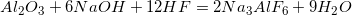The rate law for this reaction is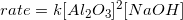.

What is the rate constant for this reaction if the reaction proceeds at an initial rate of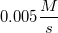when all of the reactants have initial concentrations of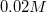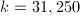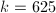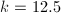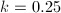Explanation:

Since the rate law is provided for the reaction, we can plug in the values that are relevant to the rate of the reaction. This will allow us to determine the rate constant.Note that HF is not included; the reaction must be zero order with respect to HF.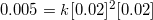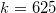### Example Question #1 : Reaction Rate And Rate Law

Consider the following reaction and experimental data.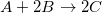Trial [A] (M) [B] (M) Initial reaction rate (M/s) 1 0.02 0.03 0.005 2 0.02 0.09 0.005 3 0.04 0.09 0.020

What is the rate law for this reaction?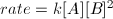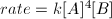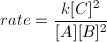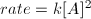Explanation:

When determining the rate law for a reaction, you need to determine the order for each reactant in the reaction. This can only be accomplished by performing an experiment where different trials show how the initial reaction rate changes based on the initial concentrations of the reactants. By keeping one initial reactant concentration constant and changing the concentration of the other reactant, we can see how the initial rate changes for each reactant.

For reactant A, we notice that the rate quadruples when its concentration is doubled by comparing trials 2 and 3. The concentration of A increases from 0.02 to 0.04, causing the rate to change from 0.005 to 0.020. This means that the order for reactant A is 2.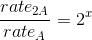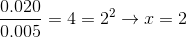For reactant B, we see that the rate does not change when the initial concentration of B is tripled by comparing trials 1 and 2. As a result, the reaction is 0 order wih respect to B.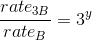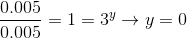Now that we have the orders for each reactant, we can write the rate law accordingly.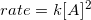Since anything to the 0 power is 1, B is omitted from the rate law and can be considered to be equal to 1.

### Example Question #2 : Reaction Rates And Reaction Order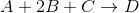You want to find the order of each reactant by manipulating the reactant concentrations in multiple trials. The table below shows the effect of altered reactant concentrations on initial reaction rate.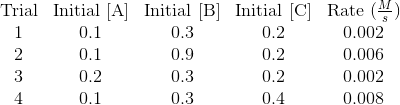Using the above trials, write the rate law for the reaction.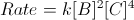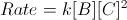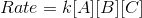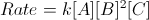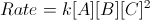Explanation:

Keep in mind that the chemical equation and its coefficients have NOTHING to do with the rate law. The order of each reactant must be determined by experiment.

To find the order of each reactant, compare the initial reaction rates of two trials in which only one of the three reactants' concentrations is altered. For example, trials 1 and 4 keep A and B equal, but C is doubled. When C is doubled, we see that the initial reaction rate is quadrupled. As a result, we determine that the order of reactant C is 2. When the reactant is altered, but the initial reaction rate is kept constant, as seen in trials 1 and 3 with respect to A, the order of that reactant is 0. Finally, when the reactant is multiplied by the same factor that the initial reaction rate is multiplied, as seen in trials 1 and 2 with respect to B, the order of the reactant is 1.

Putting the data together: A is zeroth order, B is first order, and C is second order. Our rate law can thus be written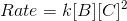.

### Example Question #1 : Reaction Rate And Rate Law

Given the following equation (2A+B --> 3C). Which of the following correctly displays the rate of the reaction?

I. -Δ[A]/2Δt

II. Δ[B]/Δt

III. Δ[C]/3Δt

II and III only

II only

I and III only

I, II, and III

I only

I and III only

Explanation:

The rate based on concentration is related to the coefficients in front of the compounds. Based on the reactants the rate should be negative (because change in concentration for the forward reaction will be negative) and based on the products should be positive. This means that II is incorrect. The rate for each compound in the reaction should be divided by it's coefficient to make it all related to 1M, showing that I and III are correct.

### Example Question #1 : Reaction Rate And Rate Law

For the following reaction H2 + 2 ICl → 2 HCl + I2 describe the rate of consumption
of H2 in regards to the consumption of ICl and the production of I2

Half of ICl, half of I2

Same as ICl, same as I2

Same as ICl, half of I2

Half of ICl, same as I2

Half of ICl, same as I2

Explanation:

By the stoichiometry, since 1 mole of of H2 and 2 moles of ICl produces 2 moles of HCl
and 1 mole of I2 , we know that H2 is consume half as fast as ICl and produced at the same
rate as I2 .

### Example Question #10 : Reaction Rate And Rate Law

Which of the following is a classic example of a first-order reaction?

A change in temperature